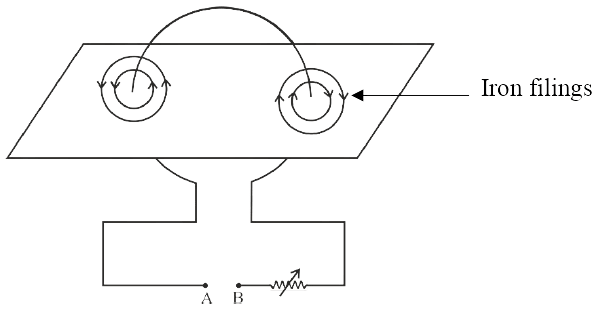# Solved 2023 Question Paper ICSE Class 10 Physics

## SECTION A (40 Marks)

#### Question 1(i)

Clockwise moment produced by a force about a fulcrum is considered to be:

1. Positive
2. Negative
3. Zero
4. None of these

Negative

Reason — If the effect on the body is to turn it clockwise, moment of force is called the clockwise moment and it is taken as negative, while if the effect on the body is to turn it anticlockwise, moment of force is called the anticlockwise moment and it is taken as positive.

#### Question 1(ii)

When the speed of a moving object is doubled, then its kinetic energy:

1. remains the same
2. decreases
3. is doubled
4. becomes four times

becomes four times

Reason — According to the formula :

Kinetic energy = $\dfrac{1}{2}$mv2

Hence, when speed (v) is doubled the kinetic energy increases by 4 times.

#### Question 1(iii)

The energy conversion in a washing machine is from ...............

1. magnetic to electrical
2. electrical to mechanical
3. electrical to magnetic
4. magnetic to electrical

electrical to mechanical

Reason — In a washing machine, electrical energy changes into mechanical energy.

#### Question 1(iv)

Which of the following radiations suffer maximum deflection in a magnetic field?

Reason — Beta radiations suffer maximum deflection in a magnetic field.

#### Question 1(v)

Speed of blue light in water is:

1. more than green light
2. more than orange light
3. more than violet light
4. more than red light

more than violet light

Reason — Speed of violet light is minimum and speed of red light is maximum. Hence, speed of blue light in water is more than violet light.

#### Question 1(vi)

A concave lens produces only ............... image.

1. real, enlarged
2. virtual, enlarged
3. virtual, diminished
4. real, diminished

virtual, diminished

Reason — A concave lens always forms a virtual and diminished image.

#### Question 1(vii)

When a body vibrates under a periodic force, the vibrations of the body are always:

1. natural vibrations
2. damped vibrations
3. forced vibrations
4. resonant vibrations

forced vibration

Reason — When a body vibrates under a periodic force, the vibrations of the body are always forced vibration.

#### Question 1(viii)

Two notes are produced from two different musical instruments, such that they have same loudness and same pitch. The produced notes differ in their:

1. Waveform
2. Frequency
3. Wavelength
4. Speed

Waveform

Reason — Different instruments produce different subsidiary notes. A note played on piano has a large number of subsidiary notes, while the same note played on violin does not contain so many subsidiary notes. Hence, they have different waveforms.

#### Question 1(ix)

When a current I flows through a wire of resistance R for time t then the electrical energy produced is given by:

1. I2Rt
2. IR2t
3. IRt
4. IRt2

I2Rt

Reason — When a current I flows through a wire of resistance R for time t then the electrical energy produced is given by I2Rt.

#### Question 1(x)

Choose the correct relation for e.m.f. (ε) and terminal voltage V:

1. ε = V [always]
2. V > ε [always]
3. V < ε [when the cell is in use]
4. None of these

V < ε [when the cell is in use]

Reason — When a cell is used to send current to an external circuit, it's terminal voltage V is less than it's e.m.f.

Hence, terminal voltage < e.m.f.

#### Question 1(xi)

If the strength of the current flowing through a wire is increased, the strength of the magnetic field produced by it:

1. decreases
2. increases
3. remains the same
4. first increases then decreases

increases

Reason — The strength of magnetic field produced can be increased by increasing the current.

#### Question 1(xii)

Specific latent heat of a substance:

1. is directly proportional to the mass
2. is directly proportional to the change in the temperature
3. depends on the material
4. is inversely proportional to the mass

depends on the material

Reason — Specific latent heat of a substance depends on the material.

#### Question 1(xiii)

Specific heat capacity of a substance X is 1900 Jkg-1°C-1 means:

1. Substance X absorbs 1900 J for 1°C rise in temperature
2. 1 kg of substance X absorbs 1900 J heat for 1°C rise in temperature
3. 1 kg of substance X absorbs 1900 J heat to increase the temperature
4. 1 kg of substance X absorbs 1900 J heat to cool down by 1°C

1 kg of substance X absorbs 1900 J heat for 1°C rise in temperature

Reason — Specific heat capacity of a substance X is 1900 Jkg-1°C-1 means 1 kg of substance X absorbs 1900 J heat for 1°C rise in temperature

#### Question 1(xiv)

When a ray of light travels normal to the given surface, then the angle of refraction is:

1. 180°
2. 90°
3. 45°

Reason — The ray of light incident normally on a plane glass slab passes undeviated, ( i.e. such a ray suffers no bending at the surface). Thus, if angle of incidence ∠i = 0°, then the angle of refraction ∠r = 0°.

#### Question 1(xv)

Small air bubbles rising up a fish tank appear silvery when viewed from some particular angle is due to the:

1. reflection
2. refraction
3. dispersion
4. total internal reflection

total internal reflection

Reason — Small air bubbles rising up a fish tank appear silvery when viewed from some particular angle because of the phenomenon of total internal reflection.

#### Question 2(i)

(a) When does the nucleus of an atom tend to become radioactive?

(b) Name a single pulley in which displacement of load and effort is not the same.

(c) State one advantage of this pulley.

(a) The nucleus of an atom becomes radioactive when number of neutrons in the nucleus exceeds the number of protons in it.

(b) Single fixed pulley.

(c) Advantage of this type of pulley is that we can lift a heavier load with a small effort.

#### Question 2(ii)

(a) What is the position of centre of gravity of a triangular lamina?

(b) When this triangular lamina is suspended freely from any one vertex, what is the moment of force produced by its own weight in its rest position?

(a) The centre of gravity for a triangular lamina is at the point of intersection of the medians.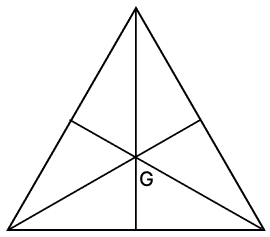(b) When the triangular lamina is suspended freely from any one vertex, the moment of force produced by its own weight in its rest position is zero. This is because the center of gravity of the triangular lamina is at the point where its medians intersect and the triangle is in rotational equilibrium. Hence, we get zero moment of force.

#### Question 2(iii)

The diagram shows wheel O pivoted at point A. Three equal forces F1, F2 and F3 act at point B on the wheel.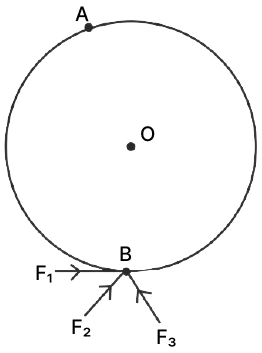(a) Which force will produce maximum moment about A?

(a) Force F1 produces the maximum moment.

(b) Moment of force = force x perpendicular distance from the point of application of the force to the pivot point.

As the three forces are equal in magnitude, the magnitude of moment of force will depend on the perpendicular distance from the point of application of the force to the pivot point.

The perpendicular distance from the line of action of F1 to the pivot point A is equal to the diameter of the wheel, while the perpendicular distance from line of action of F2 and F3 is less than the diameter, therefore, the moment produced by F1 will be greater than the moments produced by F2 and F3.

#### Question 2(iv)

(a) What should be the angle between the direction of force and the direction of displacement, for work to be negative?

(b) Name the physical quantity obtained using the formula $\dfrac{\text{U}}{\text{h}}$, where U is the potential energy and h is the height.

(a) 180°

(b) As gravitational potential energy [U] = mgh

Hence, $\dfrac{U}{h}$ = mg

Therefore, the physical quantity obtained is weight of the object.

#### Question 2(v)

Calculate the power spent by a crane while lifting a load of mass 2000 kg, at velocity of 1.5 ms-1. (g = 10 ms-2).

Given,

mass = 2000 kg

velocity = 1.5 ms-1

weight or downward force = mg = 2000 x 10 = 20,000 N

From formula, P = F x v

P = 20,000 x 1.5 = 30,000 W = 30 kW

Hence, power spent by the crane = 30 kW.

#### Question 2(vi)

A metal foot ruler is held at the edge of a table. It is pressed at its free end and then released. It vibrates.

(a) Name the vibrations produced.

(b) State one way to increase the frequency of these vibrations.

(a) The vibrations produced are known as free vibrations or natural vibrations.

(b) If we place the ruler partially off the table then a shorter length of the ruler is free to vibrate, hence the frequency of vibration increases. The shorter the length of the ruler, the higher the frequency of its natural vibrations.

#### Question 2(vii)

'A geyser is rated 240 W – 220 V'. Explain the meaning of this statement.

It means that if the geyser is operated on a 220 V supply, the electric power consumed by it is 240 W i.e., 240 J of electric energy is consumed by the geyser in 1 s.

#### Question 3(i)

(a) Is it possible for a concave lens to form an image of size two times that of the object? Write Yes or No.

(b) What will happen to the focal length of the lens if a part of the lens is covered with an opaque paper?

(a) No.

(b) The focal length of the lens will not change.
Reason — The focal length of a lens is a characteristic property of the lens itself and is determined by its shape and refractive index. Covering a portion of the lens with opaque paper will not alter the lens's inherent optical properties.

#### Question 3(ii)

(a) Which electrical component protects the electric circuit in case of excess current and which can also be used as a switch?

(b) Name the wire to which this electrical component is connected in an electric circuit.

(a) Miniature circuit breaker [MCB] can be used to protect the electric circuit in case of excess current and can also be used as a switch.

(b) The MCB is always connected in series with the live wire in the household circuit.

#### Question 3(iii)

A copper conductor is placed over two stretched copper wires whose ends are connected to a D.C. supply as shown in the diagram.

(a) What should be the magnetic poles at the points A and B lying on either side of the conductor to experience the force in the upward direction?

(b) Name the law used to find these polarities.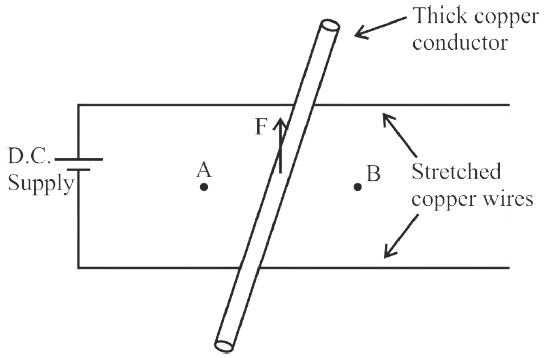(a) The magnetic poles at the points A and B should be of opposite polarity for the conductor to experience the force in the upward direction. So, if point A is North pole then point B should be South pole and vice versa.

(b) Fleming's left hand rule can be used to find these polarities.

#### Question 3(iv)

Thermal capacities of substances A and B are same. If mass of A is more than mass of B then:

(a) Which substance will have more specific heat capacity?

(b) Which substance will show greater rise in temperature if the same amount of heat is supplied to both?

(a) B will have more specific heat capacity.
Reason

$\text{Specific heat capacity (c)} = \dfrac{\text{Heat Capacity of body (C')}}{\text{mass of body (m)}} \\[1em] \therefore \text{Specific heat capacity (c)} ∝ \dfrac{1}{\text{mass}}$

Specific heat capacity of B will be more as its mass is less.

(b) Both substances will show equal rise in temperature as their thermal capacities are the same.

#### Question 3(v)

The radioactivity of a radioactive isotope is not affected by chemical changes. This is because radioactivity is a nuclear phenomenon specifically related to the stability of the isotope's nucleus, while chemical changes involve the rearrangement of electrons and the formation or breaking of chemical bonds.

## SECTION B (40 Marks)

#### Question 4(i)

The diagram below shows the ray OP travelling through an equilateral prism of a certain material.

(a) Calculate the value of i2, if the angle of deviation is 43°.

(b) What is the ray QS called?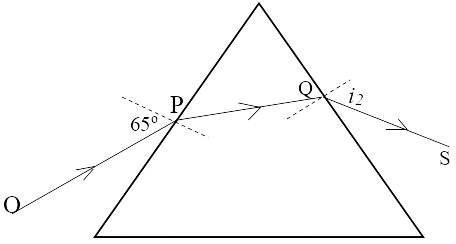(a) Given,

angle of prism (A) = 60° for an equilateral prism.

angle of incidence (i1) = 65°

Angle of deviation (δ) = 43°

𝑒 = i2 = ?

From formula,

Angle of deviation (δ) = 𝑖 + 𝑒 − 𝐴

Substituting the values we get:

43 = 65 + i2 - 60°

i2 = 43 - 5 = 38°

Hence, i2 = 38°

(b) Emergent ray

#### Question 4(ii)

Copy the diagram given below and complete the path of the light ray PQ, as it emerges out of the prism by marking necessary angles. The critical angle of glass is 42°.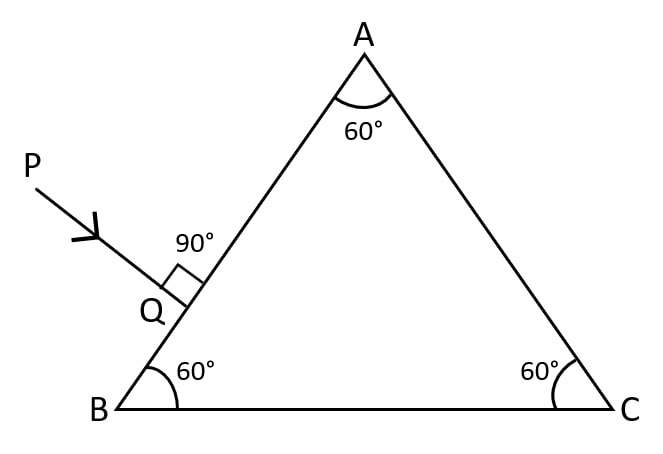Below is the completed diagram showing the path of the light ray till it emerges out of the prism with all angles marked: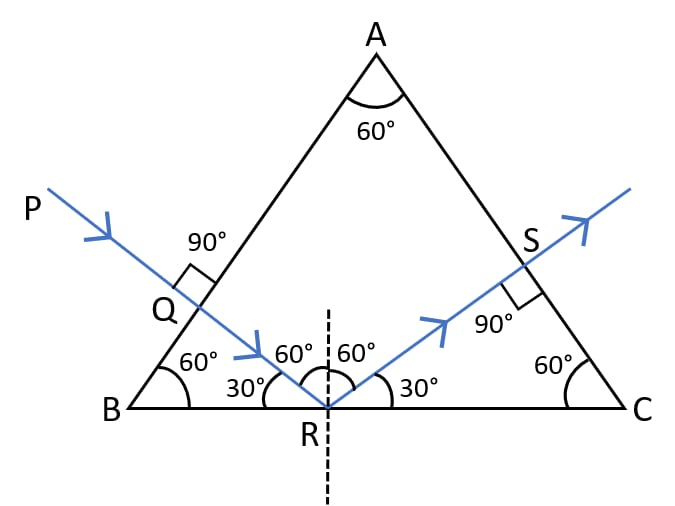#### Question 4(iii)

The diagram below shows two parallel rays A (Orange) & B (Blue) incident from air, on air-glass boundary.

(a) Copy and complete the path of the rays A and B.

(b) How do the speeds of these rays differ in glass?

(c) Are the two refracted rays in glass parallel? Give a reason.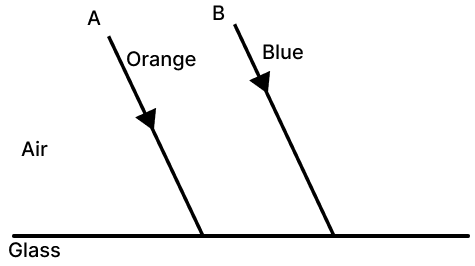(a) Ray B (Blue) will bend more than ray A (Orange) as shown in the diagram below: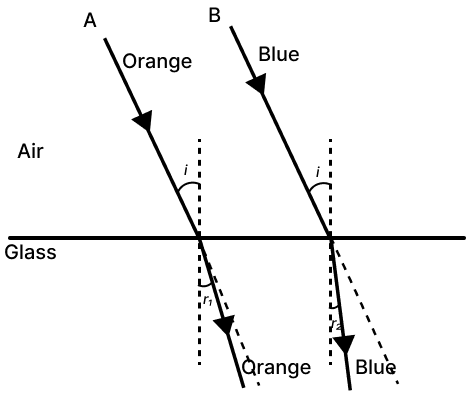(b) In glass, speed of orange (A) light is more than that of blue light (B).

(c) The two refracted rays are not parallel in glass as orange light with more speed bends less while blue light with slow speed bends more.

#### Question 5(i)

A convex lens of focal length 10 cm is placed at a distance of 60 cm from a screen. How far from the lens should an object be placed so as to obtain a real image on the screen?

Given, focal length = 10 cm

Image distance (v) = 60 cm

Object distance (u) = ?

From lens formula —

$\dfrac{1}{v} - \dfrac{1}{u} = \dfrac{1}{f} \\[1em]$

Substituting the values in the formula we get,

$\dfrac{1}{60} - \dfrac{1}{\text{u}} = \dfrac{1}{10} \\[1em] \Rightarrow \dfrac{1}{60} - \dfrac{1}{10} = \dfrac{1}{\text{u}} \\[1em] \Rightarrow \dfrac{1 - 6}{60} = \dfrac{1}{\text{u}} \\[1em] \Rightarrow \dfrac{-5}{60} = \dfrac{1}{\text{u}} \\[1em] \Rightarrow \dfrac{1}{\text{u}} = \dfrac{-1}{12} \\[1em] \Rightarrow u = -12$

∴ The object should be placed at a distance of 12 cm in front of the lens.

#### Question 5(ii)

(a) A coin kept inside water [µ=$\dfrac{4}{3}$] when viewed from air in a vertical direction appears to be raised by 3.0 mm. Find the depth of the coin in water.

(b) How is the critical angle related to the refractive index of a medium?

(a) Given, µ=$\dfrac{4}{3}$

Shift = 3.0 mm

We know,

refractive index = $\dfrac{\text{real depth}}{\text{apparent depth}}$

$\dfrac{4}{3}$ = $\dfrac{\text{x}}{\text{apparent depth}}$

apparent depth = $\dfrac{\text{3x}}{4}$

Shift = real depth - apparent depth

$3 = \text{x} - \dfrac{\text{3x}}{4} \\[1em] \Rightarrow 3 = \dfrac{\text{4x - 3x}}{4} \\[1em] \Rightarrow 3 = \dfrac{\text{x}}{4} \\[1em] \Rightarrow \text{x} = 4 \times 3 = 12$

∴ Depth of coin in water = 12 mm

(b) Critical angle [C] = sin-1 $\dfrac{1}{μ}$

#### Question 5(iii)

(a) Infrared radiations are used in warfare. Explain with reason, why.

(b) A ray of light is incident at 45° on an equilateral prism in the diagram below.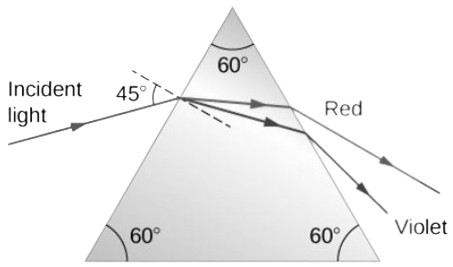1. Name the phenomenon exhibited by the ray of light when it enters and emerges out of the prism.
2. State the cause of the above phenomenon mentioned by you.

(a) Infrared radiations are used for signals during war because they are not visible and they are not absorbed much in the medium.

(b)

1. Dispersion.
2. The cause of dispersion of white light is the change in speed of light with wavelength.
When white light passes through a glass prism, its constituent colours (red, orange, yellow, green, blue, indigo, violet) travel with different speeds in the prism because each colour has different wavelength. This causes the dispersion of light.

#### Question 6(i)

A block and tackle system of pulleys has velocity ratio 4.

(a) Draw a labelled diagram of the system indicating clearly, the direction of the load and the effort.

(b) What is the value of the mechanical advantage of the given pulley system if it is an ideal pulley system?

(a) Labelled diagram of the block and tackle system of pulleys with velocity ratio 4 is shown below: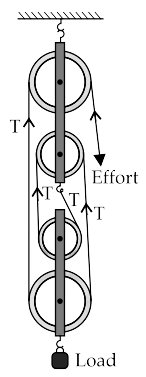(b) The load is being supported by five segments of the string. Therefore, L = 5T and E = T

∴ M.A. = $\dfrac{\text{load}}{\text{effort}}$ = $\dfrac{5\text{T}}{\text{T}}$ = 5

#### Question 6(ii)

A metre scale of weight 50 gf can be balanced at 40 cm mark without any weight suspended on it.

(a) If this ruler is cut at its centre then state which part [0 to 50 cm or 50 to 100 cm] of the ruler will weigh more than 25 gf.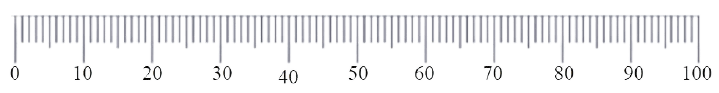(b) What minimum weight placed on this metre ruler can balance this ruler when it is pivoted at its centre?

(a) Given, wt of scale = 50 gf

Let wt. from [0 to 40] cm be W1 and [40 to 100] cm be W2

W1 + W2 = 50

⇒ W1 = 50 - W2 .....(1)

In equilibrium,

Anti-clockwise moment = Clockwise moment

(40 - 0) × W1 = (100 - 40)W2

40W1 = 60W2

40 [50 - W2] = 60W2 [.....From (1)]

40 x 50 - 40W2 = 60W2

2000 = 60W2 + 40W2

2000 = 100W2

W2 = $\dfrac{2000}{100}$

W2 = 20 gf

Now, W1 = 50 - 20 = 30 gf

∴ [0 to 40 cm] part of ruler weighs 30 gf.

Hence, the part [ 0 to 50 cm] of the ruler will weigh more 25 gf.

(b) Given the metre scale balances at 40 cm mark, so its weight of 50 gf acts at 40 cm mark.

Its weight of 50 gf produces anticlockwise moment about the centre.

Let the minimum weight needed be m.

In equilibrium,

Anti-clockwise moment = Clockwise moment

50 x (50 - 40) = m x (100 - 50)

⇒ 50 x 10 = m x 50

⇒ m = $\dfrac{50 \times 10}{50}$

⇒ m = 10 gf

Hence, minimum weight needed to balance the metre ruler when it is pivoted at its centre = 10 gf.

#### Question 6(iii)

A car of mass 120 kg is moving at a speed 18 km/h and it accelerates to attain a speed of 54 km/h in 5 seconds. Calculate:

(a) the work done by the engine.

(b) the power of the engine.

(a) Given,

Initial velocity (vi) = 18 km/h

Converting km/h to m/s

18 x $\dfrac{5}{18}$ = 5 m/s

Final velocity (vf) = 54 km/h

Converting km/h to m/s

54 x $\dfrac{5}{18}$ = 15 m/s

Work done = Change in kinetic energy = $\dfrac{1}{2}$m(vf2 - vi2)

= $\dfrac{1}{2}$ x 120 x (152 - 52)

= $\dfrac{1}{2}$ x 120 x (225 - 25)

= 60 x 200 = 12000 J

Hence, work done = 12000J

(b) Power = $\dfrac{\text{work done}}{\text{time}}$ = $\dfrac{12000}{5}$ = 2400 watt.

Hence, power of engine = 2400 watt.

#### Question 7(i)

(a) Which characteristic of sound is affected due to the larger surface of a school bell?

(b) Calculate the distance covered by the Ultrasonic wave having a velocity of 1.5 kms-1 in 14 s, when it is received after reflection by the receiver of the SONAR.

(a) Loudness.

(b) Given,

Velocity = 1.5 kms-1

Time = 14 sec

Distance = $\dfrac{\text{velocity} \times \text{time} }{2}$

(As the wave travels to the target and back so distance covered will be half of the distance travelled by the wave.)

Substituting values in the above formula we get,

Distance covered = $\dfrac{1.5 \times 14}{2}$

= 1.5 x 7 = 10.5 km

Hence, distance covered by the Ultrasonic wave = 10.5 km.

#### Question 7(ii)

(a) Complete the following nuclear changes:

23892P ⟶ Q + 42He ⟶ R + 0-1e

(b) Name the nuclear radiation which has the highest ionizing power.

(a) 23892P ⟶ 23490Q + 42He ⟶ 23491R + 0-1e

(b) Alpha (α) radiations have the highest ionizing power.

#### Question 7(iii)

We are able to see the T.V. channels clearly when we set T.V. on auto-tuning.

(a) Which phenomenon led to the clear visibility of the channels, due to auto tuning?

(b) Define the above phenomenon mentioned by you.

(c) Give any one more example of this phenomenon.

(a) Resonance.

(b) When the frequency of the externally applied periodic force on a body is equal to its natural frequency, the body readily begins to vibrate with an increased amplitude. This phenomenon is known as resonance.

(c) Air in sound box vibrates with higher amplitude due to vibrating string of musical instruments. This is an example of resonance.

#### Question 8(i)

(a) Define specific resistance.

(b) What happens to the specific resistance of a conductor if its length is doubled?

(c) Name a substance whose specific resistance remains almost unchanged with the increase in its temperature.

(a) Specific resistance of a material is the resistance of a wire of that material of unit length and unit area of cross section.

(b) Specific resistance of the wire remains unchanged since it does not depend on the dimensions of the wire. It is the characteristic property of the substance.

(c) Manganin.

#### Question 8(ii)

(a) Which nuclear radiation will travel undeviated in an electric field?

(b) How can one stop the radiations escaping from a nuclear reactor in a nuclear power plant?

(c) Name one internal source of background radiations.

(b) The nuclear reactor of the power plant must be shielded with thick lead and steel walls.

(c) Radioactive substances such as potassium (K-40), carbon (C-14) and radium present inside our body.

#### Question 8(iii)

Find the value of current I drawn from the cell.

(a) Calculate the current I.

(b) Calculate the terminal voltage.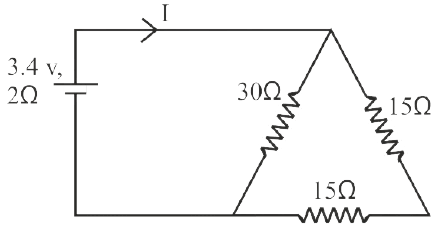(a) As, the two 15Ω resistors are in series

So, Rs = 15 + 15 = 30Ω

Now, Rs (i.e., 30Ω) and 30Ω are in parallel,

$\therefore \dfrac{1}{\text{R}_p} = \dfrac{1}{30} + \dfrac{1}{30} \\[1em] = \dfrac{2}{30} \\[1em] = \dfrac{1}{15}$

∴ Rp = 15Ω

Now, total resistance of the circuit = 2Ω + 15Ω = 17Ω

$\text{I} = \dfrac{\text{emf}}{\text{total resistance}} \\[1em] = \dfrac{3.4}{17} \\[1em] = 0.2 \text{ A}$

∴ Current I = 0.2 A

(b) Terminal voltage [V] = emf - Ir
= 3.4 - [0.2 x 2] = 3.4 - 0.4
= 3 V

Hence, terminal voltage = 3 V

#### Question 9(i)

Calculate the total amount of heat energy required to melt 200 g of ice at 0°C to water at 100°C.

(Specific latent heat of ice = 336 J g-1, specific heat capacity of water = 4.2 J g-1°C-1)

Given,

m = 200 g

specific heat capacity of water = 4.2 J g-1 K-1

specific latent heat of ice = 336 J g-1

total amount of heat energy required = ?

Heat energy taken by ice at 0° C to convert into water at 0° C
= m x L
= 200 × 336
= 67200 J

Heat energy taken by water to raise the temperature from 0° C to 100° C
= m x c x change in temperature
= 200 × 4.2 × (100 - 0)
= 200 × 4.2 × 100
= 84000 J

Total heat energy gained is
= 67200 + 84000
= 1,51,200 J

Hence, total amount of heat energy required = 1,51,200 J

#### Question 9(ii)

(a) State the principle of calorimetry.

(b) Name the material used for making a calorimeter.

(c) Write one characteristic property of the material chosen for making a calorimeter.

(a) When a hot body is brought in contact with a cold body, then heat lost by the hot body is equal to the heat gained by the cold body, provided there is no heat loss in the environment.

(b) Thin sheets of copper.

(c) The specific heat capacity of the material should be very low.

#### Question 9(iii)

The diagram below shows a cardboard on which iron filings are kept. A wire bent in the form of a loop is seen passing through the cardboard. When current flows through it the iron filings arrange themselves as shown below.

(a) State the polarities of the battery at A and B.

(b) State the effect on the magnetic field if an iron rod is held along the axis of the coil.

(c) State one way to:

1. Change the polarity of the coil.
2. Decrease the strength of the magnetic field around the coil.